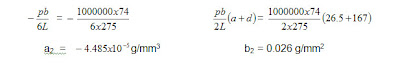## Tuesday, November 15, 2011

### Experiment on Hydrostatic PressureTheory of experiment

The hydrostatic force on any surface is due to the fluid pressure acting on that surface. Pressure is a normal stress which is positive when in compression. Since the pressure is everywhere normal to the surface, the resultant pressure force (Fp) is also normal to the surface. The magnitude of Fp is

where p = gysin a, g = specific weight of the fluid, y = distance measured from level of zero pressure and measured in the plane of the surface, and a = angle which the plane of the surface makes with the horizontal.

Partial Immersion :# Objective of experiment

To determine the center of pressure on a partially submerged plane surface.

# Apparatus

Hydraulics Bench F1-10 and Hydraulics Pressure Apparatus F1-12.

# Procedure

1. The quadrant has placed on the two dowel pins and used the clamping screw, fasten

to the balance arm.

2. Measured a, L, depth d and width b of the quadrant end face.

3. The balance arm have positioned on the knife edges (pivot) with the Perspex tank on

the bench.

4. The balance pan have hanged from the end of the balance arm.

5. A length of hose is connected from the drain cock to the sump and a length from the

bench feed to the triangular aperture on the top of the Perspex tank.

6. The tank has leveled by used the adjustable feet and spirit level.

7. The counter balance weight is moved until the balance arm is horizontal.

8. Closed the drain cock and admitted water until the level reaches the bottom edge of

the quadrant. Placed a weight on the balance pan, slowly adding water into the tank

until the balance arm is horizontal.

9. The water level on the quadrant and the weight on the balance pan have recorded.

10.Fine adjustment of the water level can be achieved by overfilling and slowly draining

by used the stop cock.

11.The above for each increment of weight has repeated until the water level reaches the

top of the quadrant end face.

12.Removed each increment of weight noting weights and water levels until weights have

been removed.

# Results and calculations

 FILLING TANK DRAINING TANK AVERAGE Weight, m(g) Height of water, y(mm) Weight, m(g) Height of water, y(mm) m y y2 m/y2 50 70 90 115 150 42.5 51 58.5 66.5 77.5 50 70 90 115 150 42.5 51 58.5 66.5 77.5 50 70 90 115 150 42.5 51 58.5 66.5 77.5 1806.25 2601 3422.25 4422.25 6006.25 0.028 0.027 0.026 0.026 0.025

The measurement for the equipment ;

a = 26.5 mm, b = 74 mm, d = 167 mm, L = 275 mm

Plot m/y2 vs y graph. The slope of this graph should be – pb/6L and the intercept should be pb/2L (a+d) . The – pb/6L and pb/2L (a+d) is theory part.

Experiment Part

Let a1 = slope of the graph let b1 = intercept for the graph

= - 0.0015/20 = 0.039 g/mm2

= - 7.5 x 10-5 g/mm3

Theory PartSince the theory and experiment result are different, it should to find out the percentage different between them by using following formulas :Discussion of results

From the results obtained, the slope for the graph is -7.5 x 10-5 g/mm3 and the intercept is 0.039g/mm2. However for the theoretical result for the slope is -4.485 x 10-5g/mm3 and the intercept is 0.026 g/mm2. For the normal situation, the slope and the intercept for experiment part should be same with the theoretical part. If even there are different, but if the different percentage is not more than 10 %, the results still can be accepted.

For this experiment, after obtained the different percentage between the theoretical part and experiment part, is found that the different for the slope between both side is 67.22% and the different for the intercept between both side are 50%. So that the different between both site is more than 10 %, this lab results not acceptable.

There are so much differences between theoretical and experimental part caused by human error. This can be occurred because when taking the reading, it only use eyes look at the reading directly and not by some apparatus which is more accuracy. This laboratory results out of the range from normal situation also caused by mistake when filling water and draining water from the tank.

Conclusion

From the experiment done, it is a way to determine centre of pressure. This laboratory is considered not well but laboratory test sometimes will not success. It may caused by many reasons.